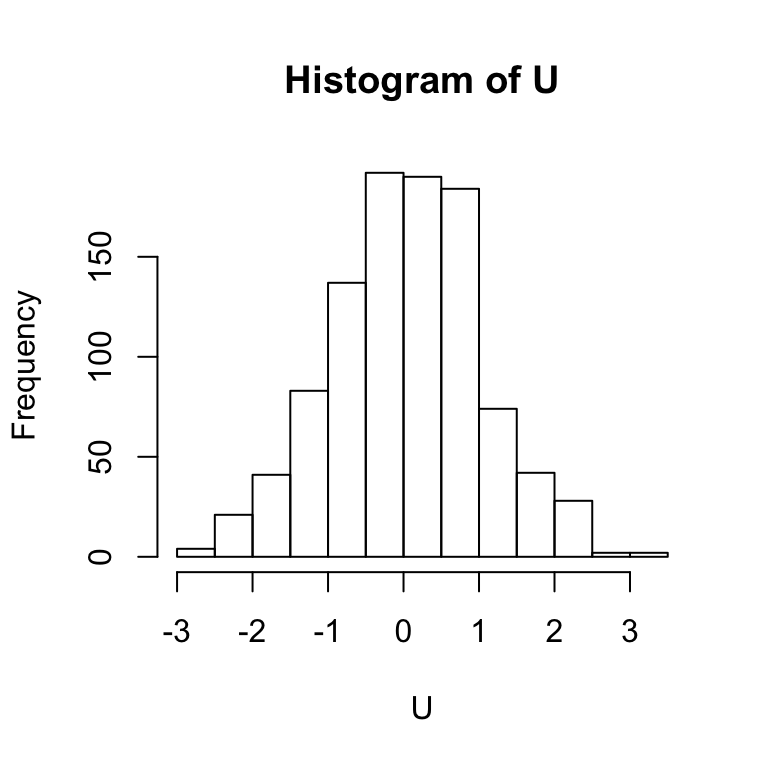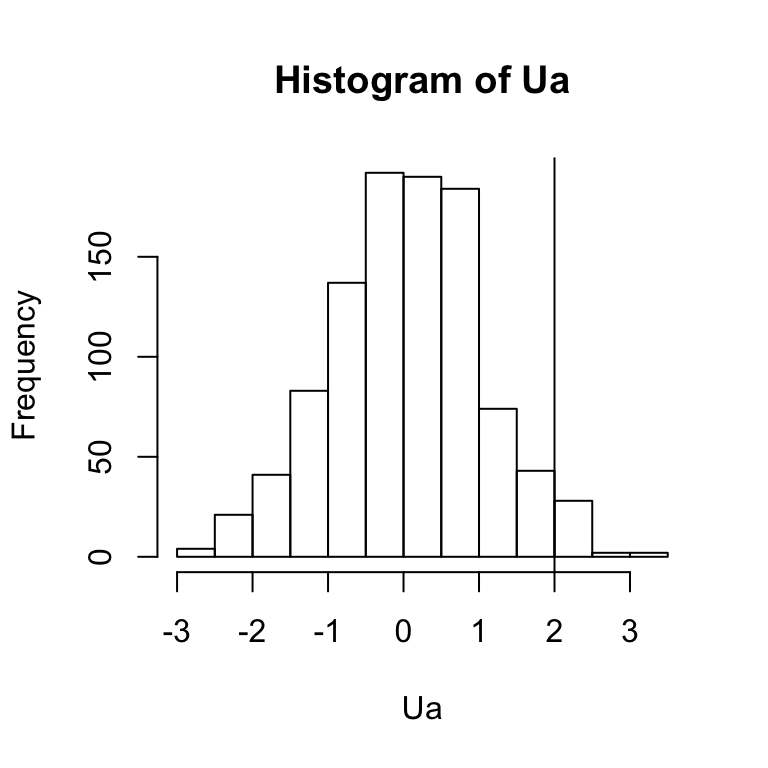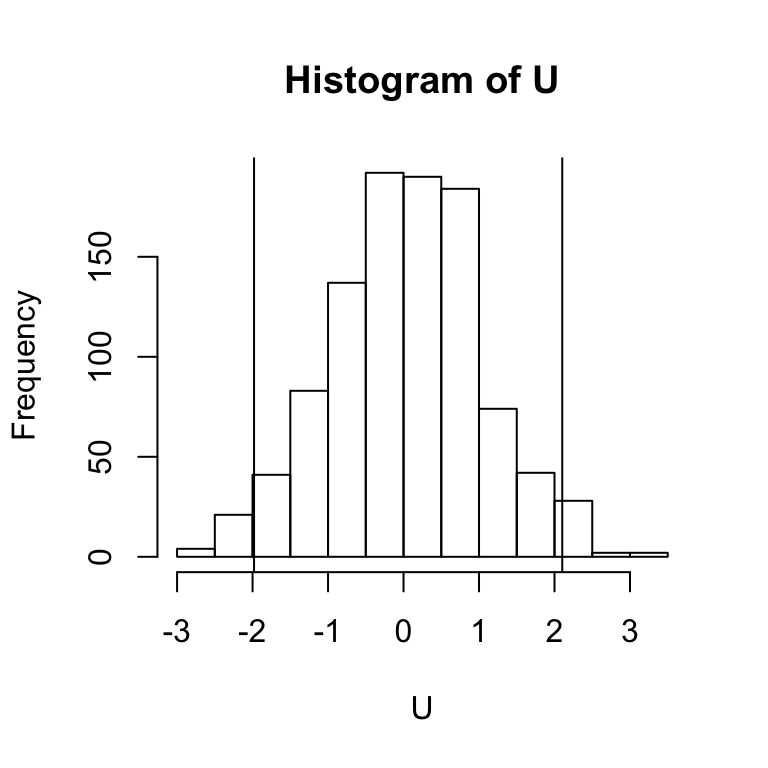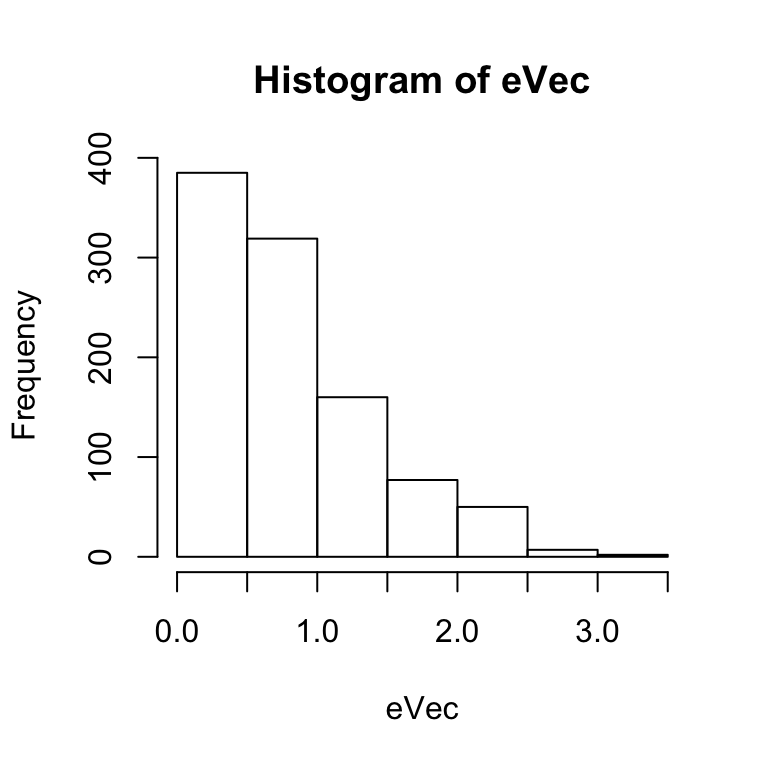# 1 Introduction

This tutorial offers an introduction to conformal inference, which is a method for constructing valid (with respect to coverage error) prediction bands for individual forecasts. The appeal of conformal inference is that it relies on few parametric assumptions. For formal treatments of conformal inference, refer to the following:

• Shafer and Vovk (2007) “A Tutorial on Conformal Prediction”, arxiv.

• Lei et al. (2017) “Distribution-Free Predictive Inference For Regression”, arxiv.

The current tutorial is meant to be a gentle introduction to complement these more complete treatments. It follows Lei et al. very closely—sort of a “Cliffs Notes” with some worked illustrations along the way.

The code that I have written below is not meant to be production level. If you actually want to use conformal methods, then you should use the conformalInference package (github link). The code here is just to provide algorithmic illustrations of conformal methods.

# 2 Basic Idea

Suppose a sample of $$n$$ iid draws from $$P$$, $$U(n) = U_1,...,U_n \sim P$$. Consider this sample’s distribution:

set.seed(12345)
n <- 1000
U <- rnorm(n)
hist(U)Now suppose a $$n+1$$th draw, $$U_{n+1}$$, is to be taken from $$P$$. What is the probability, with respect to the sample of $$N+1$$ draws from $$P$$, that $$U_{n+1}$$ would exceed some value $$u$$? Given that all draws are iid, we can work with the empirical quantiles of the augmented sample, $$(U(n), u)$$ to see what mass resides to the right of $$u$$. E.g., suppose that $$u = 2$$. Then our augmented sample, with the associated finite sample probability, would be as follows:

u <- 2
Ua <- c(U, u)
hist(Ua)
abline(v=u)print(round(mean(Ua > u), 3))

 0.032

Suppose we set a miscoverage (“error”) rate of $$\alpha$$. E.g., suppose that we choose $$\alpha = 0.05$$. Then, we could do a line search of $$u$$ values to define an interval, $$C$$, for which $$\text{Pr}[u \in C] \ge 1-\alpha = 0.95$$.

nEval <- 200
u.candidate <- seq(from=min(U), to=max(U), length = nEval)
Cbounds <- c(u.candidate[match(5, apply(as.matrix(u.candidate),
1,
function(x){floor(2.5+100*(mean(c(U, x) < x)))}))],
rev(u.candidate)[match(95, rev(apply(as.matrix(u.candidate),
1,
function(x){ceiling(-2.5+100*(mean(c(U, x) < x)))})))])
print(Cbounds)

 -1.980157 2.102782

hist(U)
abline(v=Cbounds)This interval, $$C$$ is a conformal interval.

# 3 Naive Conformal Regression Prediction Bands

This section and the next follow Lei et al. (2017).

Now suppose that we observe $$n$$ iid draws of $$Z_i = (X_i, Y_i)$$ from $$P$$, and we can to construct a prediction band for $$Y_{n+1}$$ given $$X_{n+1}$$, also drawn from $$P$$. A naive approach would first estimate a regression function $$\mu(X_i)$$ for $$Y$$ conditional on $$X$$, generate a prediction $$\widehat{\mu}(X_{n+1})$$ and then construct a $$1-\alpha$$ band around $$\widehat{\mu}(X_{n+1})$$ using $$\pm(1-\alpha/2)$$ quantile of the distribution of fitted residuals $$|Y_i - \widehat{\mu}(X_i)|$$:

X <- rnorm(n)
Y <- X + U
regData <- data.frame(X,Y)
fitlm <- lm(Y~X, data=regData)
eVec <- abs(fitlm\$residuals)
hist(eVec)plot(X,Y)
Xnew <- -1.5
abline(fitlm)
abline(v=Xnew, lty="dashed")
muHat <- predict(fitlm, newdata=data.frame(X=Xnew))
points(Xnew, muHat, pch=19, col="red")
C.X <- c(muHat-quantile(eVec, .975), muHat+quantile(eVec, .975))
points(rep(Xnew, 2), C.X, type="l", col="red", lwd=2)